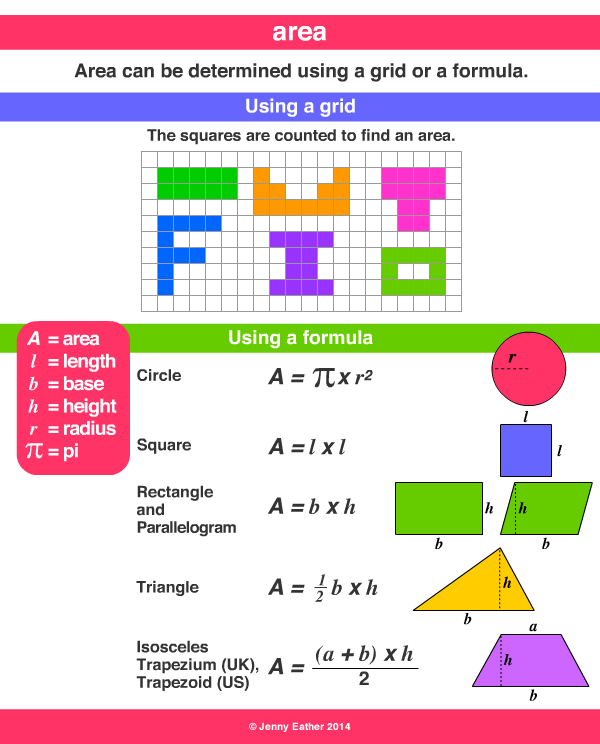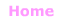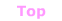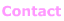Aa Bb Cc Dd Ee Ff Gg Hh Ii Jj Kk Ll Mm Nn Oo Pp Qq Rr Ss Tt Uu Vv Ww Xx Yy Zz

Aa

area

• the size a surface takes up.
• area is measured in square units.

EXAMPLES:Metric Units ~ Area

1 square metre = 10 000 square centimetres
1 hectare = 10 000 square metres
1 square kilometre = 100 hectares

Abbreviations
square centimetre cm2 square metre m2
hectare ha square kilometre km2

Imperial/Customary Units ~ Area

144 square inches = 1 square foot
9 square feet = 1 square yard
4,840 square yards = 1 acre
640 acres = 1 square mile© Jenny Eather 2014. All rights reserved.